Question

Write the linear function f with the values f(1) = 1 and f(-3) = 17. (Note: Use f(x) instead of y.) Show work to get full credits.

1.Given:

f(1) = 1 and f(-3) = 17

To find:

The lines function f.

Solution:

If f(a) = b, it means the function f passes through (a,b).

Here, f(1) = 1, it means the function f passes through (1,1).

f(-3) = 17, it means the function f passes through (-3,17).

If a linear function passes through two point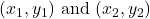, then the equation of line is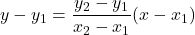The linear function f passes through (1,1) amd (-3,17). So, the equation of linear function is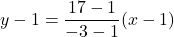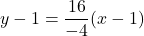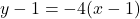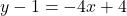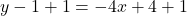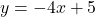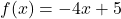Therefore, required lines function is.